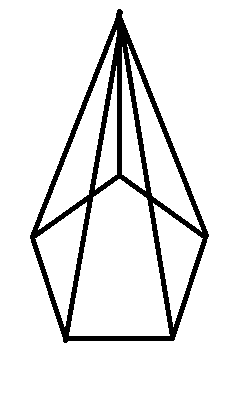The faces of a pentagonal pyramid consists ofA.One pentagon and five trianglesB.One pentagon and five rectanglesC.Two pentagon and five triangles D.Two pentagon and five rectanglesVerified
146.7k+ views
Hint: Lets first draw the pentagonal pyramid for a clear understanding and from that we can see that its base is a pentagon and the other faces are triangles.

Step 1:
Lets first draw the pentagonal pyramidStep 2 :
From the picture we can see that the pentagonal pyramid consists of six faces
Here the base is a pentagon and we can see that the remaining five faces are triangles .
In geometry, a pentagonal pyramid is a pyramid with a pentagonal base upon which are erected five triangular faces that meet at a point.
Therefore the faces of the pentagonal pyramid consists of one pentagon and five triangles .

The correct option A.

Note: Pentagonal pyramid is a pyramid with a pentagonal base upon which are erected five triangular faces that meet at a point
Two pentagonal pyramids can be attached to each other to form the pentagonal bipyramid
A pentagonal pyramid can be attached to a dodecahedron to form an augmented dodecahedron